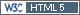## Compilation [Study As­sess­ment]

Dear all,

in R / `lmerTest()` one can get maximum likelihood estimation by setting the argument `REML=FALSE` in the model statement (default is `REML=TRUE`). Satterthwaite’s DF are 10.858 for the intercept and 6.884 for the slope. In `lme()` use `method="ML"` (default is `method="REML"`). Below a compilation (in analogy to BE rounded to four decimal figures and grouped by “similarity”):

```Software        Method      Estimate      90% CI    ═══════════════════════════════════════════════════ SAS (Smith)   ML/Satter.   B0 1.94    1.54   2.35                              B1 0.7615  0.679  0.844  SAS           ML/Satter.   B0 1.9425  1.5391 2.3458                            B1 0.7615  0.6789 0.8441 R lmerTest    ML/Satter.   B0 1.9425  1.5391 2.3458                            B1 0.7615  0.6789 0.8441 ─────────────────────────────────────────────────── R lme         ML           B0 1.9425  1.5175 2.3675                            B1 0.7615  0.6738 0.8491 ─────────────────────────────────────────────────── SAS           ML/contain.  B0 1.9414  1.5175 2.3675                            B1 0.7615  0.6738 0.8491 ─────────────────────────────────────────────────── SAS           REML/Satter. B0 1.9414  1.4968 2.3860                            B1 0.7617  0.6696 0.8539 PHX/WNL       REML/Satter. B0 1.9414  1.4968 2.3860                            B1 0.7617  0.6696 0.8539 R lmerTest    REML/Satter. B0 1.9414  1.4968 2.3860                            B1 0.7617  0.6696 0.8539 ─────────────────────────────────────────────────── NCSS          method?      B0 1.9414  1.4849 2.3978                            B1 0.7617  0.6659 0.8576 ─────────────────────────────────────────────────── SAS           ML/contain.  B0 1.9414  1.4807 2.4020                            B1 0.7617  0.6665 0.8570 R lme         REML         B0 1.9414  1.4807 2.4020                            B1 0.7617  0.6665 0.8570 ─────────────────────────────────────────────────── PHX/WNL       REML/resid.  B0 1.9414  1.4515 2.4313                            B1 0.7617  0.6665 0.8570```

My preference is REML/Satterthwaite because one could reproduce results in three different software packages. I would avoid NCSS; no idea how the calculation is done.

Cheers,
Helmut SchützThe quality of responses received is directly proportional to the quality of the question asked. ☼
Science QuotesIng. Helmut Schütz